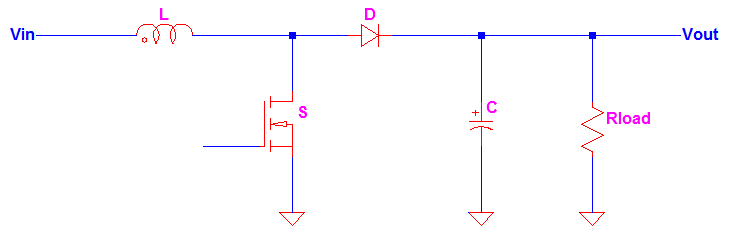# How to Calculate the Duty Cycle of Boost Converter

The inductor waveform is the key on how to calculate the duty cycle of boost converter. You can get direct equation for boost converter duty cycle formula from different sites but here I will discuss how it is derived. Meanwhile, a familiar boost converter schematic is shown in Figure 1.

The inductor of the boost converter charges during Ton and discharges during Toff. The inductor current waveform is then resembled like Figure 2 below.

Figure 2 is an inductor waveform of a continuous conduction mode boost converter. A boost converter by the way can operate in three modes; continuous conduction (CCM), discontinuous conduction (DCM) and boundary mode. For CCM the inductor current will not reach zero after every switching cycle. For DCM, the inductor current is reaching zero before the next Ton occur. On the other hand for the boundary mode, the inductor current is just

zero when the next Ton happens.

The duty cycle of CCM and boundary mode is the same. However, you cannot use this duty cycle for DCM boost converter. But in practical application, DCM operation is avoided because in this region the peak current is high and the RMS losses are high as well. It may demand for high rating devices. Apart from these, DCM may introduce problem in EMC. Based on experienced, a boost converter is usually set to CCM at higher loads and at full load. When the load decreases on the other hand, the operation may enter DCM but this time the current is already very small and the danger of device stress is no longer an issue.

How to Calculate the Duty Cycle of Boost Converter – Analysis during Ton

During Ton, the switch S is on. The diode will be reversed biased and this

time the load is being supplied by the energy stored in the capacitor C. The inductor will charge. The flow of the current is from Vin to inductor L then to switch S. By KVL,Then expressing VLEq. 1

(Note: VL is the voltage of the inductor and VSw is the turn on voltage of the switch.)

The voltage across inductor is also defined by below formula.Eq. 2

Expressing the change in current diEq. 3

Substitute Eq.1 to Eq. 3Eq. 4

Then we will get rid of the variable dt by integrating the equation from 0 to Ton.Eq. 5

How to Calculate the Duty Cycle of Boost Converter – Analysis during Toff

During Toff, the inductor reverses its polarity and the diode at this time will be forward biased and the load will be supplied by the energy in the inductor. The energy on the inductor will start to decay. The flow of current is from Vin to diode D then to the load. Applying KVL,Expressing VL,Eq. 6

(Note: VL is the voltage on the inductor and this is not a drop during this time since it is reversing its polarity. VF is the forward voltage of the diode.)

Substituting [Eq. 6] to [Eq. 3] will result toEq. 7

Then we will eliminate the variable dt through integration from Ton to T.Eq. 8

Now go back to Figure 2, the magnitude of the inductor current during Ton and Toff is equal. Therefore we can equate [Eq. 5] and [Eq. 8].Then substitute Ton = DT(Note: D is the boost converter duty cycle which is equal to the ratio of on time to the entire signal period.)

Then solving for the duty cycle, DEq. 9

Assuming ideal values (diode and switch voltage drops are zero)Eq. 10

The above duty cycle is can be used also with the boundary mode. However it cannot anymore applicable to DCM. DCM duty cycle derivation is different since it has already a dead time and the integration limit during Toff is not anymore from Ton to T. Anyway, as mentioned above, the practical operation of a boost converter is CCM at higher loads.

If someone asking you how to calculate the duty cycle of boost converter, you can provide answer already!

You may be interested to

Boost Converter Component Selection Template and Boost Converter Component Stress Calculation Template.